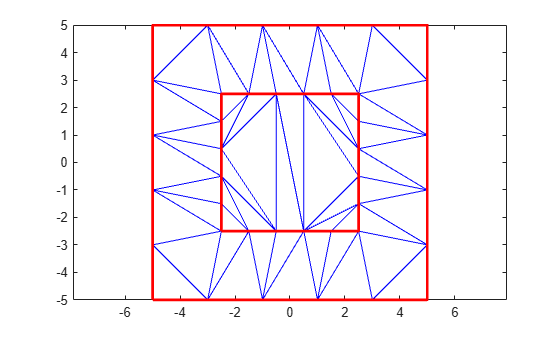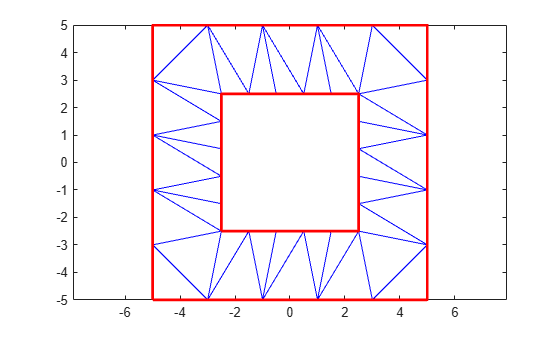# isInterior

Query points inside Delaunay triangulation

## Syntax

``TF = isInterior(DT)``

## Description

example

````TF = isInterior(DT)` returns a column vector of logical values that indicate whether the triangles in a 2-D constrained Delaunay triangulation are inside a bounded geometric domain. An element of `TF` is 1 (`true`) when the corresponding triangle in `DT` is inside the domain and 0 (`false`) otherwise.```

## Examples

collapse all

Compute and plot the triangles of a 2-D constrained Delaunay triangulation within a specified boundary.

Create a geometric domain whose shape is a square frame.

```outerprofile = [-5 -5; -3 -5; -1 -5; 1 -5; 3 -5; 5 -5; 5 -3; 5 -1; 5 1; 5 3; 5 5; 3 5; 1 5; -1 5; -3 5; -5 5; -5 3; -5 1; -5 -1; -5 -3]; innerprofile = outerprofile.*0.5; P = [outerprofile; innerprofile];```

Define the edge constraints.

```outercons = [(1:19)' (2:20)'; 20 1;]; innercons = [(21:39)' (22:40)'; 40 21]; C = [outercons; innercons];```

Create the constrained Delaunay triangulation.

`DT = delaunayTriangulation(P,C);`

Plot the triangulation, highlighting the inner and outer squares in red.

```triplot(DT) hold on plot(DT.Points(innercons',1),DT.Points(innercons',2), ... '-r','LineWidth',2) plot(DT.Points(outercons',1),DT.Points(outercons',2), ... '-r','LineWidth',2) axis equal ```Plot only the triangles between the inner and outer squares, highlighting the inner and outer squares in red.

```figure TF = isInterior(DT); triplot(DT.ConnectivityList(TF,:),DT.Points(:,1),DT.Points(:,2)) hold on plot(DT.Points(outercons',1),DT.Points(outercons',2), ... '-r','LineWidth',2) plot(DT.Points(innercons',1),DT.Points(innercons',2), ... '-r','LineWidth',2) axis equal```## Input Arguments

collapse all

Constrained Delaunay triangulation, specified as a scalar 2-D `delaunayTriangulation` object with a set of constrained edges that define a bounded geometric domain. A bounded domain is a region enclosed by multiple constrained edges that do not intersect or overlap.

Data Types: `delaunayTriangulation`

## Tips

• `isInterior` can produce incorrect or inconsistent results when boundary constraints intersect or overlap. To avoid this behavior, use constraints that form one or multiple closed boundaries that do not intersect or overlap. When boundary constraints are nested without intersections or overlaps, the inside or outside status alternates across the boundaries.

## Version History

Introduced in R2013a